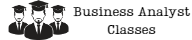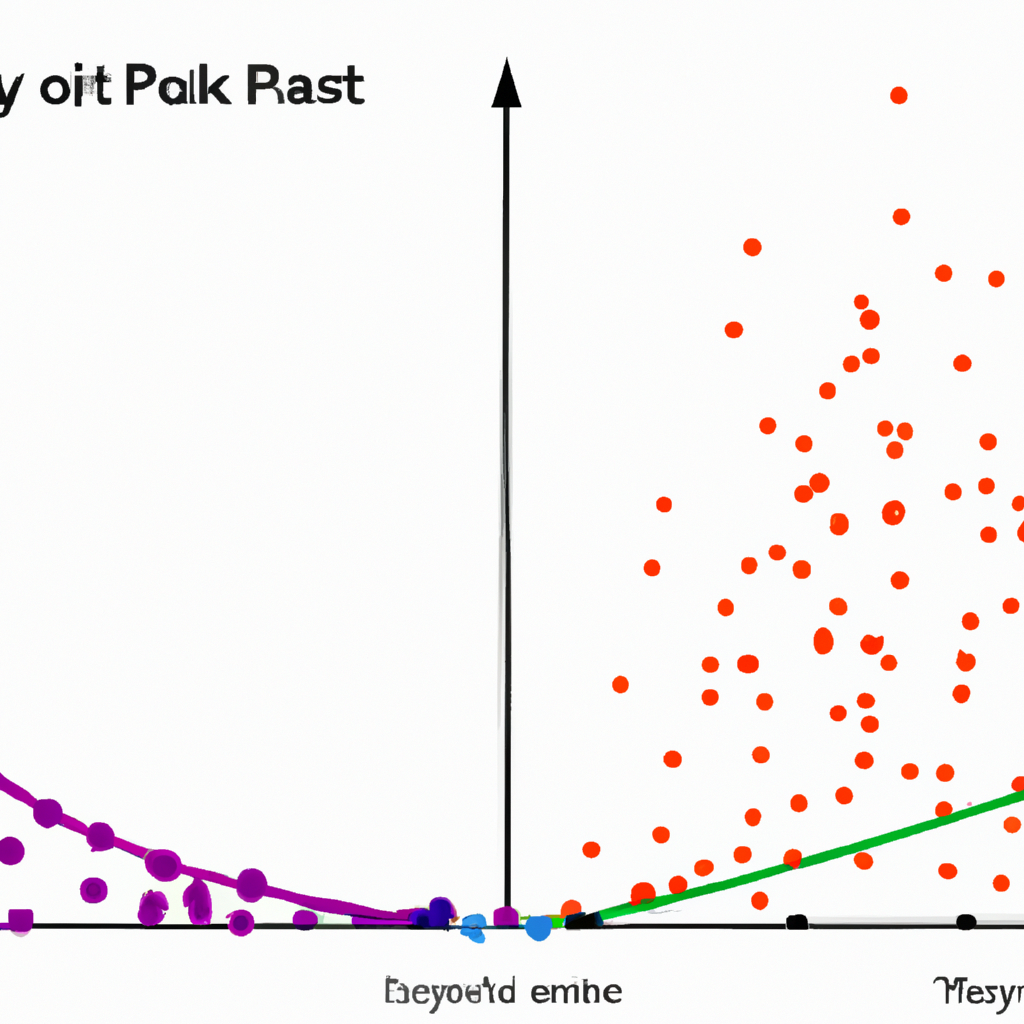Search

# The Role of Correlation Analysis in Understanding Relationships Between Variables

When it comes to understanding relationships between variables, correlation analysis is a powerful tool that can provide valuable insights. Correlation analysis is a statistical method that measures the strength and direction of the relationship between two variables. In this article, we will explore the role of correlation analysis and how it can be used to gain a better understanding of relationships between variables.## What is Correlation Analysis?

Correlation analysis is a statistical method that measures the strength and direction of the relationship between two variables. The strength of the relationship is measured by the correlation coefficient, which ranges from -1 to 1. A correlation coefficient of -1 indicates a perfect negative correlation, which means that as one variable increases, the other variable decreases. A correlation coefficient of 1 indicates a perfect positive correlation, which means that as one variable increases, the other variable also increases. A correlation coefficient of 0 indicates no correlation, which means that there is no relationship between the two variables.

## Types of Correlation

There are two types of correlation: positive correlation and negative correlation. Positive correlation occurs when the two variables move in the same direction. Negative correlation occurs when the two variables move in opposite directions.

## How Correlation Analysis is Used

Correlation analysis is used to determine the strength and direction of the relationship between two variables. It is often used in research to investigate the relationship between variables and to test hypotheses. For example, if a researcher wants to investigate the relationship between exercise and weight loss, they can use correlation analysis to determine if there is a relationship between the two variables. If there is a strong positive correlation between exercise and weight loss, then the researcher can conclude that exercise is an important factor in weight loss.

Correlation analysis provides several advantages over other statistical methods. First, it is easy to understand and interpret. The correlation coefficient is a simple measure that can be easily understood by both researchers and non-researchers. Second, it is a powerful tool for identifying relationships between variables. Correlation analysis can help researchers identify important variables that may be related to an outcome of interest. Finally, correlation analysis can be used to test hypotheses. Researchers can use correlation analysis to test hypotheses about the relationship between variables.

## Limitations of Correlation Analysis

Correlation analysis also has several limitations. First, correlation does not imply causation. Just because two variables are correlated does not mean that one variable causes the other. Second, correlation analysis is sensitive to outliers. Outliers can have a significant impact on the correlation coefficient, and researchers need to be careful when interpreting the results of correlation analysis. Finally, correlation analysis is limited to measuring linear relationships between variables. If the relationship between two variables is non-linear, then correlation analysis may not be appropriate.

## Conclusion

Correlation analysis is a powerful tool that can provide valuable insights into the relationships between variables. It is easy to understand and interpret, and it can be used to test hypotheses and identify important variables. However, researchers need to be aware of the limitations of correlation analysis and use it appropriately. By understanding the role of correlation analysis in understanding relationships between variables, researchers can gain a better understanding of the world around us.

### Try some of our classes

Enter your email and we’ll send you some samples of our favorite classes.

#### Course Categories

Quisque velit nisi, pretium ut lacinia in, elementum id enim.